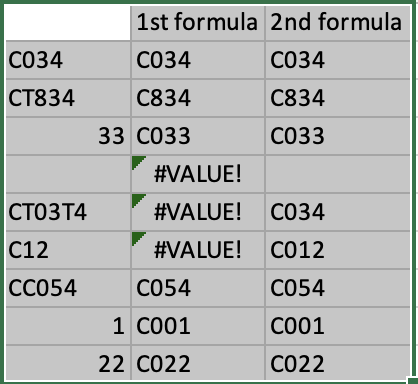asked on

# Normalizing values in cells with formula in Excel / Google Sheets

Hello all,
I thought this be trivial but I'm just not finding the right solution.

I have a column of cell entries where there are occasion input errors for the non-numerical portion of the values. So, I want to automatically correct these errors.

Examples:
Input value / Corrected input value)
CT021 / C021
044 / C044
33 / C033

In other words, the correct value is always going to be in the format, "Cxxx". Where xxx = 000 - 999. The leading zeros are important.

Thank youLast Comment
byundt

8/22/2022 - Mon

THIS SOLUTION ONLY AVAILABLE TO MEMBERS.
View this solution by signing up for a free trial.
Members can start a 7-Day free trial and enjoy unlimited access to the platform.
SOLUTION
byundt

THIS SOLUTION ONLY AVAILABLE TO MEMBERS.
View this solution by signing up for a free trial.
Members can start a 7-Day free trial and enjoy unlimited access to the platform.
Doug Van

Thank you Byundt.

So, I tested a few scenarios with the first formula:
C034  - good
C12 - error (Function UNARY_MINUS parameter 1 expects number values. But C12 is a text and cannot be coerced to a number.)
CC054 - error (same as above)
1 - result = C000
22  - result = C000

I couldn't get the second formula to work.

byundt

I am not reproducing your results.

My test data started in cell DD2, so you may need to update the second formula in two places to get it to work.Doug Van

Greetings Byundt,

Thanks again. I truly owe you again. Although this particular question was already answered for what I needed, I still thank you for providing an alternative formula that I could learn from. :)

=TEXT(TEXTJOIN("",,IFERROR(--MID(A2,(LEN(A2)-(ROUND(Len(A2)/3))),3),"")),"\C000")

Works for all the examples, including an important forgotten example: "C123".

Here is a working sheet:

If you have a moment, I am trying to figure out a solution to this problem:
Doug Van

Thank you again to both Ron and byundt. I really appreciate your help. :)
SOLUTION
byundt

THIS SOLUTION ONLY AVAILABLE TO MEMBERS.
View this solution by signing up for a free trial.
Members can start a 7-Day free trial and enjoy unlimited access to the platform.
Doug Van

Thank you again.
=ARRAY_CONSTRAIN(ARRAYFORMULA(TEXT(TEXTJOIN("",,IFERROR(--MID(A2,LEN(A2)-{2,1,0},1),"")),"\C000")), 1, 1)

I'm not sure why my alternative formula didn't work for you...
=TEXT(TEXTJOIN("",,IFERROR(--MID(A2,(LEN(A2)-(ROUND(Len(A2)/3))),3),"")),"\C000")
but if you're curious, my sheet will now open for you.

``````=TEXT(TEXTJOIN("",,IFERROR(--MID(A2,(LEN(A2)-(ROUND(Len(A2)/3))),3),"")),"\C000")